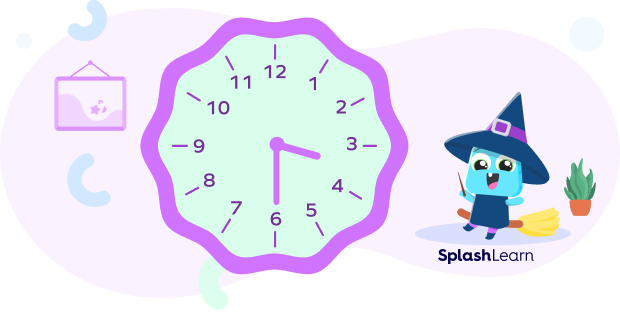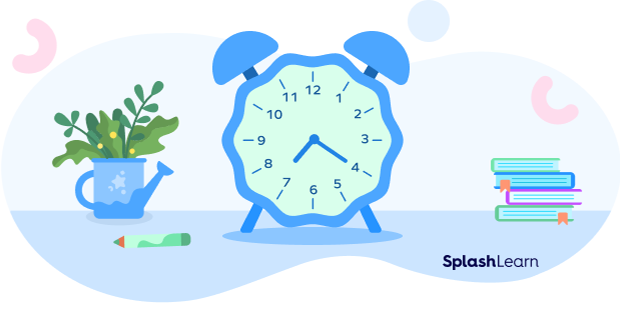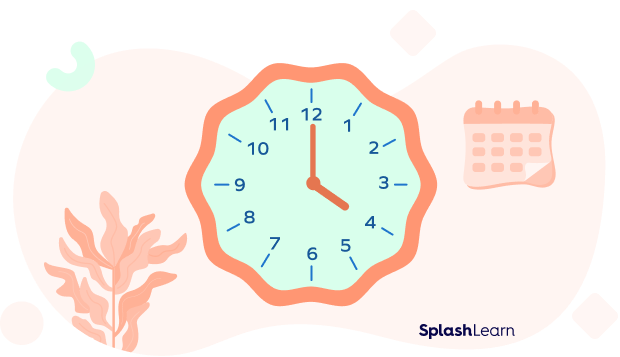# Minute in Math – Definition with Examples

Home » Math Vocabluary » Minute in Math – Definition with Examples

## What is the Minute?

There are three hands on the clock: the hour hand, the minute hand, and the second hand. The shortest hand of the clock denotes the hour, the middle hand denotes the minute, and the longest hand denotes the second. Minutes can be calculated by multiplying the number it points to by five.

Example:

In this analog clock, the hour hand, as you can see, lies at 4, and the minute hand is at 8. So multiply the number to which the minute hand points by 5 to calculate the minutes, i.e., $8 \times 5 = 40$. Therefore, the time on the clock is 4:40.

## Parts of a Clock

An analog clock is divided into 12 sections. The numbers move from 1 to 12. Between two numbers, there is a gap of five minutes.

Lastly, the most important hand is the minute hand of the clock. One hour of the clock equals 60 minutes. Let’s say, for example, your lunch break starts at 2:30 p.m. The “30” indicates the minutes, and the “2” is the hour. That is, there are thirty more minutes until three o’clock.

## How Can You Easily Tell Time?

Here are a few steps to easily tell time.

Step 1:  Observe the minute and hour hand on the clock. The hour hand is identified from its position from numbers one to twelve.

Step 2: Count the distance between the numbers for the minute hand, each being 5 minutes apart.

Step 3: Simply add all the minutes.

## How to Tell Minutes

Use multiples of five for the distance between any two digits. For example, in the picture given below, you can see the minute hand is on 2. So, $5 \times 2 = 10$. And since the hour hand points to 10, the time is 10 minutes past 10, or 10:10.

## Solved Examples

Example 1: What is the time on this clock?

●  5:00

●  12:00

●  1:00

●  10:00

Solution: The time indicated on this clock is 5:00. As the hour hand points to the number 5 and the minute hand points to 12.

Example 2: What is the time on this clock?

Solution: The time is 9:10. The minute hand points to 2, which indicates 10 minutes, and the hour hand points to 9.

Example 3: How many minutes are shown here?

Solution: As you can see, the hour hand points to 7. So, it is 7 hours. The minute hand points to 1. So, the time becomes 7:05. Hence, there are 5 minutes shown here.

## Practice Problems

1

### Indicate the time on this clock.3:00
12:30
3:30
6:15
CorrectIncorrect
The hour hand is between 3 and 4, and the minute hand points to 6. So, the time on this clock is 3:30.
2

### What time is shown on the clock?7:00
7:20
8:20
4:35
CorrectIncorrect
The hour hand is between 7 and 8, and the minute hand points to 4. So, the time on this clock is 7:20.
3

### What is the time on this clock?1:00
4:00
8:00
12:00
CorrectIncorrect
The hour hand points to 4, and the minute hand points to 12. So, the time on this clock is 4:00.

## Conclusion

Minute is the measuring unit of time. A child can be taught this concept through games and activities at SplashLearn. There are many similar concepts given on this website.